## How To Force Text String to Upper,Lower, or Proper Case in Excel

This post will guide you how to force test strings to uppercase or lowercase or Proper cases through an Excel formula or data validation in your Excel 2013/2016. How to convert text to upper, lower, or proper case in Excel.

Assuming that you want to force text strings to Upper case when you type words into one cell, and how to do it. You can use an Excel function in Data Validation feature to achieve it. Just do the following steps:

Step1: go to DATA tab, click Data Validation command under the Data Tools group. Then clicking on the Data Validation menu from the drop down menu list. Then the Data Validation window will appear.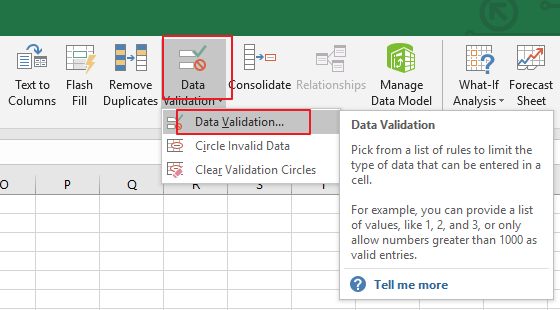Step2: in the Data Validation dialog box, and switch to Settings tab, and click the Allow drop down list and choose Custom value from the drop down menu list.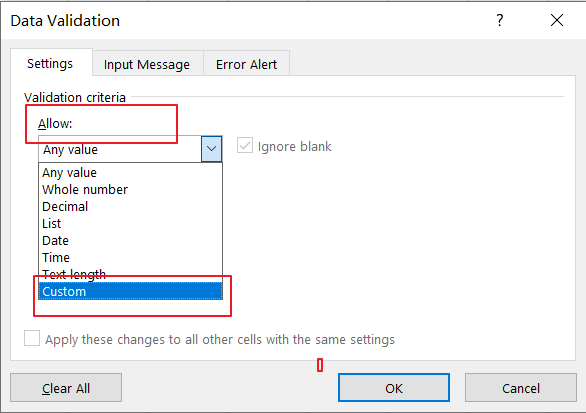Step3: Then type the following formula in the Formula text box in the Data Validation dialog box.

Note:

If you want to convert text string to Upper Case, type the following formula:

=EXACT(UPPER(B1),B1)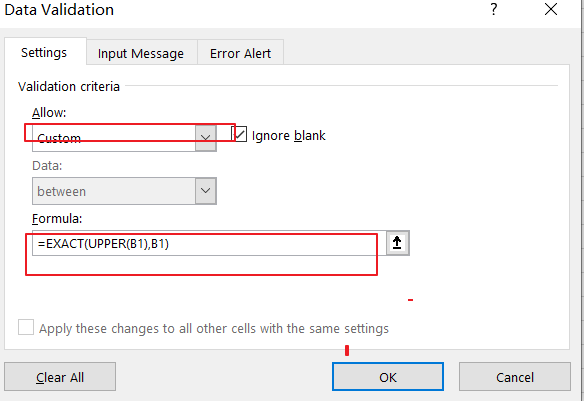If you want to convert text string to Lower Case, just use the following formula:

=EXACT(LOWER(B1),B1)

If you want to convert text string to Proper Case, just use the following formula:

=EXACT(PROPER(B1),B1)

Step4: then you need to switch to Error Alert tab in the Data Validation dialog box. And select Stop value from the Style drop down list box, and type some error message under Error message text box. Then click on Ok button.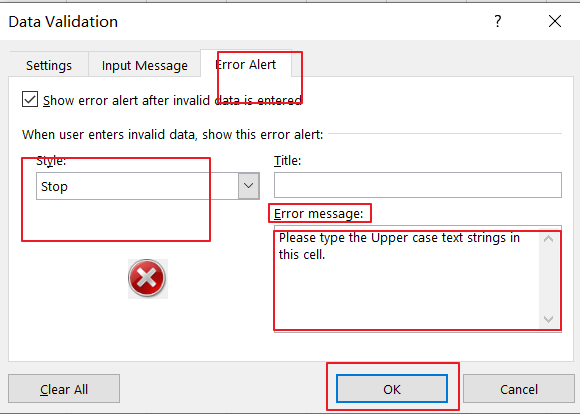Step5: you can try to enter one text string in cell B1 to verify if it take effect.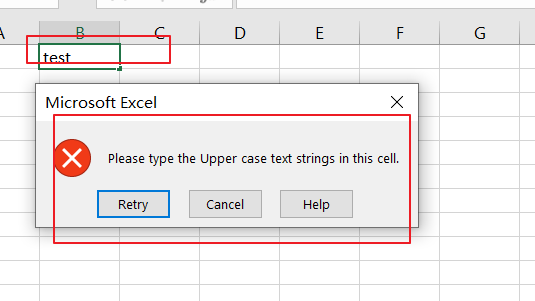You can also use an Excel VBA Macro to achieve the same result of forcing Text string to Upper, Lower or Proper cases in you worksheet. Just do the following steps:

Step1: open your excel workbook and then click on “`Visual Basic`” command under `DEVELOPER` Tab, or just press “`ALT+F11`” shortcut.Step2: then the “`Visual Basic Editor`” window will appear.

Step3: choose on your current worksheet from the left Project Explorer, and double click on it to open the code window, and the copy and paste the following VBA code into it.

For Upper Case:

```Private Sub Worksheet_Change(ByVal Target As Range)

Target.Value = VBA.UCase(Target.Value)

End Sub```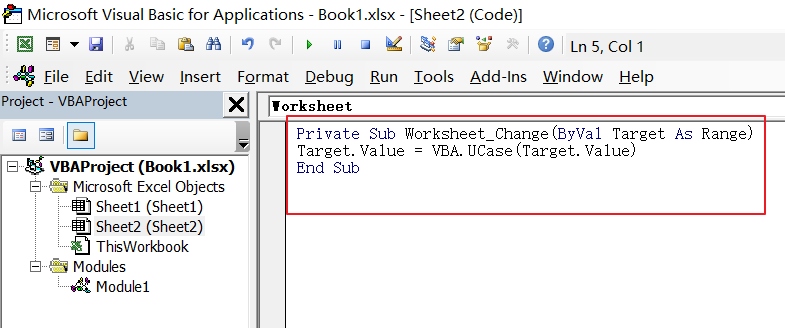For Lower Case:

```Private Sub Worksheet_Change(ByVal Target As Range)

Target.Value = VBA. LCase (Target.Value)

End Sub```

For Proper Case:

```Private Sub Worksheet_Change(ByVal Target As Range)

Target.Value = Application.WorksheetFunction.Proper(Target.Value)

End Sub```

Step4: save and close the current VBA window. And then you can try to enter one text string to verify it. when you type one word when it is lower or proper cases, and it will convert it to Uppercase.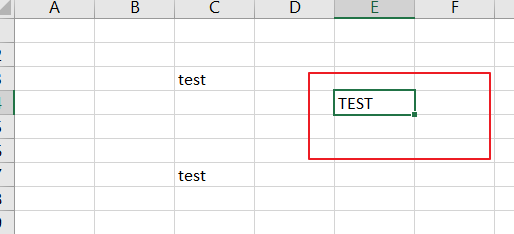### Related Functions

• Excel UPPER function
The Excel UPPER function converts all characters in text string to uppercase.The UPPER function is a build-in function in Microsoft Excel and it is categorized as a Text Function.The syntax of the UPPER function is as below:= UPPER (text)…
• Excel LOWER function
The Excel LOWER function converts all characters in a text string to lowercase.The syntax of the LOWER function is as below:= LOWER  (text)…
• Excel Proper Function
The Excel PROPER function capitalizes the first character in each word of a text string and set other characters to lowercase. The syntax of the PROPER function is as below:= PROPER  (text)…
• Excel EXACT function
The Excel EXACT function compares if two text strings are the same and returns TRUE if they are the same, Or, it will return FALSE.The syntax of the EXACT function is as below:= EXACT (text1,text2)…

## How to Check If a Cell is Uppercase, Lowercases or Capitalize in Excel

This post will guide you how to check if a cell is uppercase in Excel 2013/2016. How do I identify all letters in cells are lowercases or capitalize in Excel. How to change letters to uppercase, lowercases or capitalize first letter for all words in cells in Excel.

## Check If All Letters are Uppercase

If you want to check if all letters in cells are uppercase, you can use a formula based on the EXACT function and the UPPER function. Like this:

`=EXACT(B1,UPPER(B1))`

Type this formula into a blank cell and press Enter key to apply this formula. If All letters are uppercases, and it returns TRUE. Otherwise, it returns FALSE. Then drag the AutoFill Handle down to other cells to apply this formula.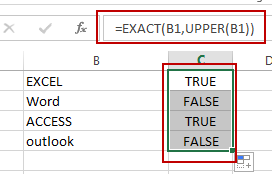Let’ see how this formula works:

The UPPER function will convert all letters to uppercase. And then pass the result to EXACT function as its second argument. And the EXACT function will compare if two values are equal. If equal, returns TRUE. Otherwise, returns FALSE.

## Check If All Letters are Lowercase

If you need to identify all characters in cells are lowercase, you can use a formula based on the LOWER function and the EXACT function. Like this:

`=EXACT(B1,LOWER(B1))`

Type this formula into cell C1, and press Enter key to apply this formula. If all letters are lowercases, and it returns TRUE. Otherwise, it returns FALSE. Then drag the AutoFill Handle down to other cells to apply this formula.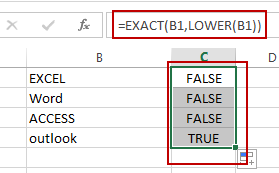The LOWER function converts all letters to lowercase.

## Check If the First Letters are capitalized

If you want to check if the first character in each word is capitalized, you can use a formula based on the EXACT function and the PROPER function. Like this:

`=EXACT(B1,PROPER(B1))`

Type this formula into cell C1, and press Enter key to apply this formula. If all the first letters are capitalized, and it returns TRUE. Otherwise, it returns FALSE. Then drag the AutoFill Handle down to other cells to apply this formula.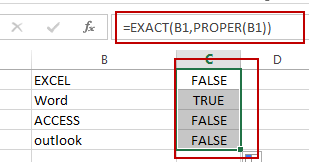The PROPER function will capitalize the first letter in each word in Cell.

### Related Functions

• Excel UPPER function
The Excel UPPER function converts all characters in text string to uppercase.The UPPER function is a build-in function in Microsoft Excel and it is categorized as a Text Function.The syntax of the UPPER function is as below:= UPPER (text)…
• Excel LOWER function
The Excel LOWER function converts all characters in a text string to lowercase.The syntax of the LOWER function is as below:= LOWER  (text)…
• Excel Proper Function
The Excel PROPER function capitalizes the first character in each word of a text string and set other characters to lowercase. The syntax of the PROPER function is as below:= PROPER  (text)…
• Excel EXACT function
The Excel EXACT function compares if two text strings are the same and returns TRUE if they are the same, Or, it will return FALSE.The syntax of the EXACT function is as below:= EXACT (text1,text2)…

## How to Capitalize First Letter of Each Word in Excel

This post will guide you how to capitalize the first letter of each word in a cell in Excel. How do I capitalize the first letter only with a formula or VBA Macro in Excel.

## Capitalize the First Letter of Each Word with Formula

Assuming that you have a list of cells with various phrases in range B1:B4 and you only want the first letter of each word is capitalized. How to achieve it. You can use a formula based on the PROPER function to achieve the result. Just like this:

`=PROPER(B1)`

Type this formula into a blank cell and press Enter key and then drag the AutoFill Handle over to other cells to apply this formula.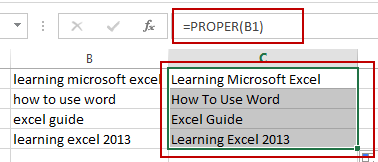You will see that the first letter of each word in cells have been capitalized.

## Capitalize the First Letter of Each Word with VBA Code

You can also use an Excel VBA macro to achieve the result of capitalizing the first letter of each word in cells. Just do the following steps:

#1 open your excel workbook and then click on “Visual Basic” command under DEVELOPER Tab, or just press “ALT+F11” shortcut.#2 then the “Visual Basic Editor” window will appear.

#3 click “Insert” ->”Module” to create a new module.#4 paste the below VBA code into the code window. Then clicking “Save” button.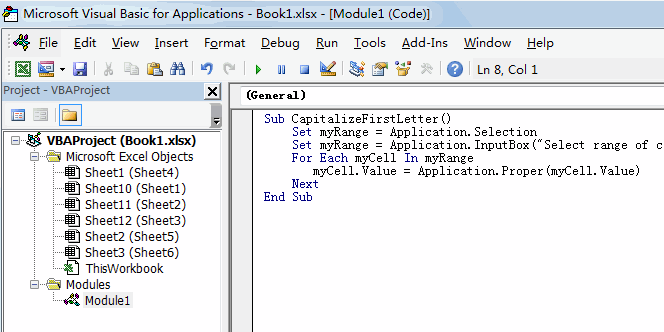```Sub CapitalizeFirstLetter()
Set myRange = Application.Selection
Set myRange = Application.InputBox("Select range of cells that you want to capitalize the first letter", "CapitalizeFirstLetter", myRange.Address, Type:=8)
For Each myCell In myRange
myCell.Value = Application.Proper(myCell.Value)
Next
End Sub```

#5 back to the current worksheet, then run the above excel macro. Click Run button.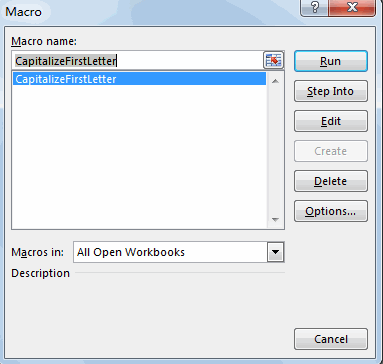#6 Select range of cells that you want to capitalize the first letter.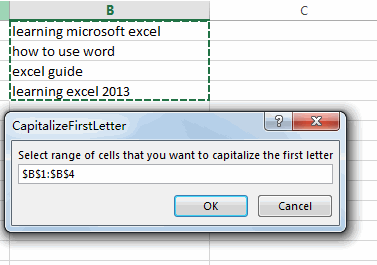#7 Let’s see the last result: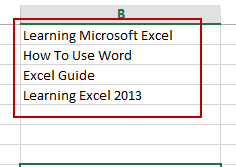Video: Capitalize the First Letter of Each Word

## Change Uppercase to Title Case or Sentence Case in Excel

This post will guide you how to change uppercase letters to sentence case with a formula in Excel. How do I change uppercase to title case in Excel. How to change Uppercase to title case or sentence case with VBA macro in Excel.

## Change Uppercase to Title Case

If you want to change uppercase letters to title case in Excel. you need to use a formula based on the PROPER function to achieve it.Just like this:

`=PROPER(B1)`

Type this formula into a blank cell C2, and press Enter key in your keyboard, and then drag the AutoFill Handle down other cells to apply this formula.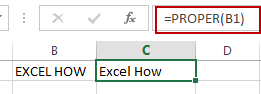You will see that All of  uppercase letters are changed to title case or proper cases.

## Change Uppercase to Title Case with VBA

You can also use an Excel VBA macro to change all uppercase letters to title cases. Just do the following steps:

#1 open your excel workbook and then click on “Visual Basic” command under DEVELOPER Tab, or just press “ALT+F11” shortcut.#2 then the “Visual Basic Editor” window will appear.

#3 click “Insert” ->”Module” to create a new module.#4 paste the below VBA code into the code window. Then clicking “Save” button.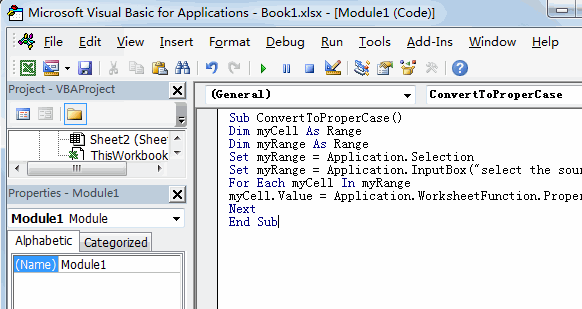```Sub ConvertToProperCase()
Dim myCell As Range
Dim myRange As Range
Set myRange = Application.Selection
Set myRange = Application.InputBox("select the source Range", "ConvertToProperCase", myRange.Address, Type:=8)
For Each myCell In myRange
myCell.Value = Application.WorksheetFunction.Proper(myCell.Value)
Next
End Sub```

#5 back to the current worksheet, then run the above excel macro. Click Run button.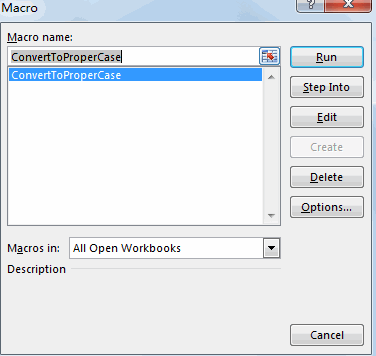#6 select one source range of cells that contain uppercase letters.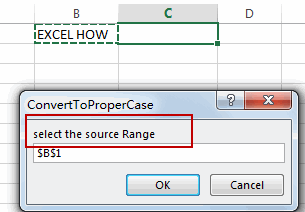#7 lets see the result.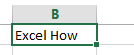### Related Functions

• Excel Proper Function
The Excel PROPER function capitalizes the first character in each word of a text string and set other characters to lowercase. The syntax of the PROPER function is as below:= PROPER  (text)…

## Change Uppercase and Lowercase Text

This post will guide you how to change the case of text to uppercase or lowercase in Excel. How do I change all uppercase text to lower case with an Excel formula.

For example, if you want to change all text string in uppercase into lowercase, you can create a formula based on the Lower function. And if you want to convert all letters in one cell into uppercase, you can use the Upper function as a formula in excel.

If you want to show in Cell C1 a lowercase version of the text in Cell B1, you can type the following formula into the formula box in Cell C1.

=LOWER(B1)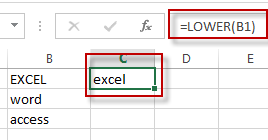If you want to show in Cell C2 an uppercase version of the text in B2, you could type the below formula into the formula box in C2.

=UPPER(B2)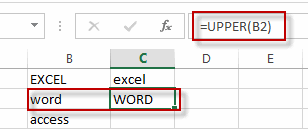If you want to show in Cell C3 a proper version of the text in B3, you could type the below formula into the formula box in C3:

=PROPER(B3)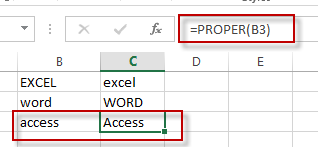### Related Functions

• Excel UPPER function
The Excel UPPER function converts all characters in text string to uppercase.The UPPER function is a build-in function in Microsoft Excel and it is categorized as a Text Function.The syntax of the UPPER function is as below:= UPPER (text)…
• Excel LOWER function
The Excel LOWER function converts all characters in a text string to lowercase.The syntax of the LOWER function is as below:= LOWER  (text)…
• Excel Proper Function
The Excel PROPER function capitalizes the first character in each word of a text string and set other characters to lowercase. The syntax of the PROPER function is as below:= PROPER  (text)…

## How to Check If the First Letter is capitalized

This post explains that how to check if the first letter in a cell is capitalized or not in excel. How to write an excel Macro to check if the first letter in a specified cell is capitalized or not. How to test if the first letter in a table cell is capitalized with VBA macro in excel. How to capitalize the first letter in a cell with Excel formula.

## Check if the first letter is or not capitalized

To check if the first letter in a text string is or not capitalized, you can write an Excel macro to achieve it. So you can follow the below steps to check the first letter:

1# click on “Visual Basic” command under DEVELOPER Tab.2# then the “Visual Basic Editor” window will appear.

3# click “Insert” ->”Module” to create a new module4# paste the below VBA code into the code window. Then clicking “Save” button.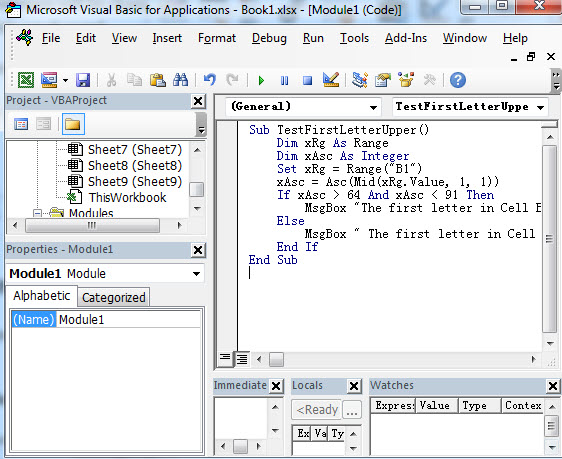```Sub TestFirstLetterUpper()
Dim xRg As Range
Dim xAsc As Integer
Set xRg = Range("B1")
xAsc = Asc(Mid(xRg.Value, 1, 1))
If xAsc > 64 And xAsc < 91 Then
MsgBox "The first letter in Cell B1 is capitalized."
Else
MsgBox " The first letter in Cell B1 is not capitalized "
End If
End Sub```

This VBA code will check if the first letter in Cell B1 is capitalized or not. So you just need to change it to other cells or range as you need.

Or you can use another VBA Macro to achieve the same result:

```Sub TestFirstLetterUpper2()
var1 = "B1"
If UCase(Left(var1, 1)) = Left(var1, 1) Then
MsgBox "First letter capitalized"
Else
MsgBox "First letter not capitalized"
End If
End Sub```

5# back to the current worksheet, then run the above excel macro.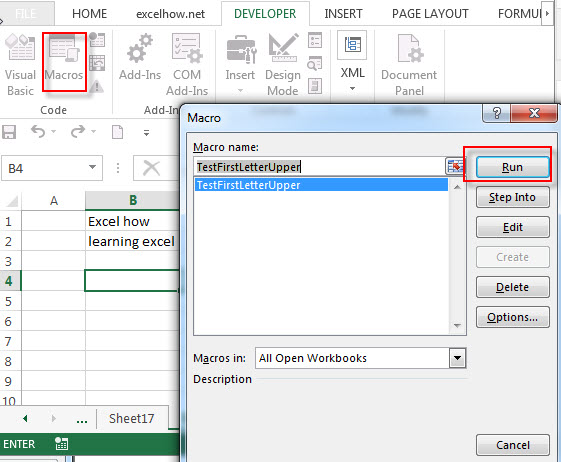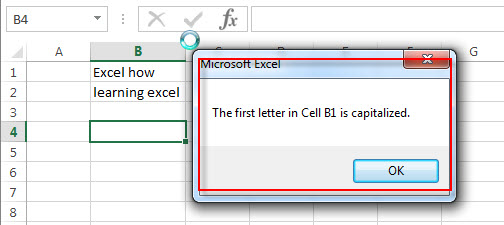## Capitalize the first letter in a Cell

Normally, it is very easy to capitalize all letters in a cell in excel, you just need to use the PROPER function to convert it.

`=PROPER(B1)`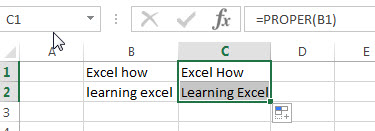If you want to capitalize only the first letter in a cell, you can create an excel formula based on the REPLACE function, the UPPER function and the LEFT function.

Assuming that you’d like to capitalize the first letter in a first word in Cell B1, then you can write down the following formula:

`=REPLACE(B1,1,1, UPPER(LEFT(B1,1)))`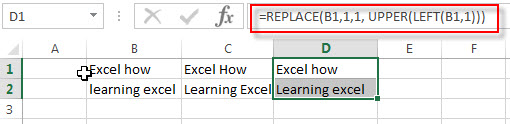You can also use another excel formula to achieve the same result as follows:

`=CONCATENATE(UPPER(LEFT(B1,1)), RIGHT(B1,LEN(B1)-1))`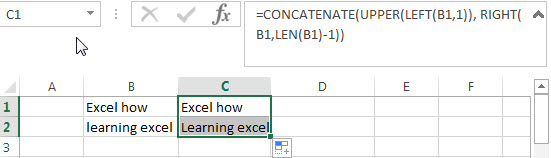### Related Functions

• Excel LEN function
The Excel LEN function returns the length of a text string (the number of characters in a text string).The LEN function is a build-in function in Microsoft Excel and it is categorized as a Text Function.The syntax of the LEN function is as below:= LEN(text)…
• Excel RIGHT function
The Excel RIGHT function returns a substring (a specified number of the characters) from a text string, starting from the rightmost character.The syntax of the RIGHT function is as below:= RIGHT (text,[num_chars])…
• Excel Replace function
The Excel DATE function returns the serial number for a date.The syntax of the DATE function is as below:= DATE (year, month, day) …
• Excel LEFT function
The Excel LEFT function returns a substring (a specified number of the characters) from a text string, starting from the leftmost character.The LEFT function is a build-in function in Microsoft Excel and it is categorized as a Text Function.The syntax of the LEFT function is as below:= LEFT(text,[num_chars])…t)…
• Excel UPPER function
The Excel UPPER function converts all characters in text string to uppercase.The UPPER function is a build-in function in Microsoft Excel and it is categorized as a Text Function.The syntax of the UPPER function is as below:= UPPER (text)…
• Excel Proper Function
The Excel PROPER function capitalizes the first character in each word of a text string and set other characters to lowercase. The syntax of the PROPER function is as below:= PROPER  (text)…
• Excel Concat function
The excel CONCAT function combines 2 or more strings or ranges together.This is a new function in Excel 2016 and it replaces the CONCATENATE function.The syntax of the CONCAT function is as below:=CONCAT (text1,[text2],…)…

## Excel Proper Function

This post will guide you how to use Excel PROPER function with syntax and examples in Microsoft excel.

### Description

The Excel PROPER function capitalizes the first character in each word of a text string and set other characters to lowercase .

The PROPER function is a build-in function in Microsoft Excel and it is categorized as a Text Function.

The PROPER function is available in Excel 2016, Excel 2013, Excel 2010, Excel 2007, Excel 2003, Excel XP, Excel 2000, Excel 2011 for Mac.

### Syntax

The syntax of the PROPER function is as below:

`= PROPER  (text)`

Where the PROPER function arguments are:
Text -This is a required argument. The text string that need to capitalize the first character in each word and the rest characters in each work will convert to lowercase.

### Example

The below examples will show you how to use Excel PROPER Text function to set the first character to uppercase in each word and set the rest characters in each word to lowercase.

#1 To proper case of the text string in B1 cell, just using formula: =PROPER (B1)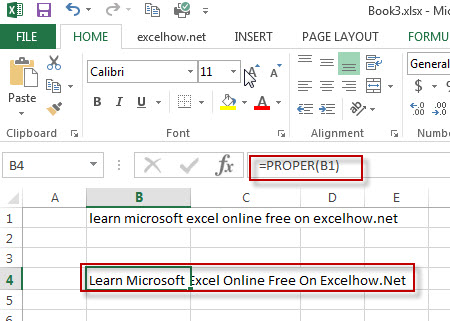### More Related PROPER Function Examples

• Change Uppercase and Lowercase Text
if you want to change all text string in uppercase into lowercase, you can create a formula based on the Lower function. And if you want to convert all letters in one cell into uppercase, you can use the Upper function as a formula in excel.…
• Change Uppercase to Title Case or Sentence Case in Excel
If you want to change uppercase letters to title case in Excel. you need to use a formula based on the PROPER function to achieve it.…